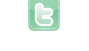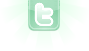This site requires JavaScript, please enable it in your browser!# VARIOUS

1
2
3
Change 50 in line 35 to 100.
line 15 you mean I forget to add mousemoved to move rocket but it does not move it remains blocked The distance is always equal to zero I have a problem of x1.getx (), x2.getx () ...
ok i erased mouseclicked because it blocks with mouseMoved it's always equal to zero but I'm looking for
I have a question The distance is calculated with respect to the point X = 0, y = 0? I just realize I thought to calculate between two points in clicking anywhere by moving the mouse, I'm wrong, Is this feasible?
ronald wrote...
The distance is calculated with respect to the point X = 0, y = 0?
Then all you need is:
`double distance = Math.hypot(x1, y1);`
hi I am looking for the mathematical formula of a projectile or rocket moving shot in vertical There are so many on Google that I do not know how to choose Thank you for your help
If moving in a straight line, no mathematical formula is needed.
yes I'm stupid I found a code on a cannon of Ubay Dillah but the missile flies straight in vertical What interests me, it is to see the missile fly vertically and not right in vertically I do not know if you understand me
In a straight line, but angled? like Missile Command?
you tilt Rocket and pull a missile that points its nose following the trajectory vertically and not standing With a round shot, you do not see but a missile starting in vertical, you see it, the missile follows the standing trajectory
So, like:
```Actor missile = new Missile();
getWorld().addObject(missile, getX(), getY());
missile.setRotation(getRotation());```
ok it's this code that I had seen at the forum thank you it works I put your code next to Ubay and removes the missile rotation and The missiles point their noses well by following the trajectory thanks again
You need to login to post a reply.
1
2
3